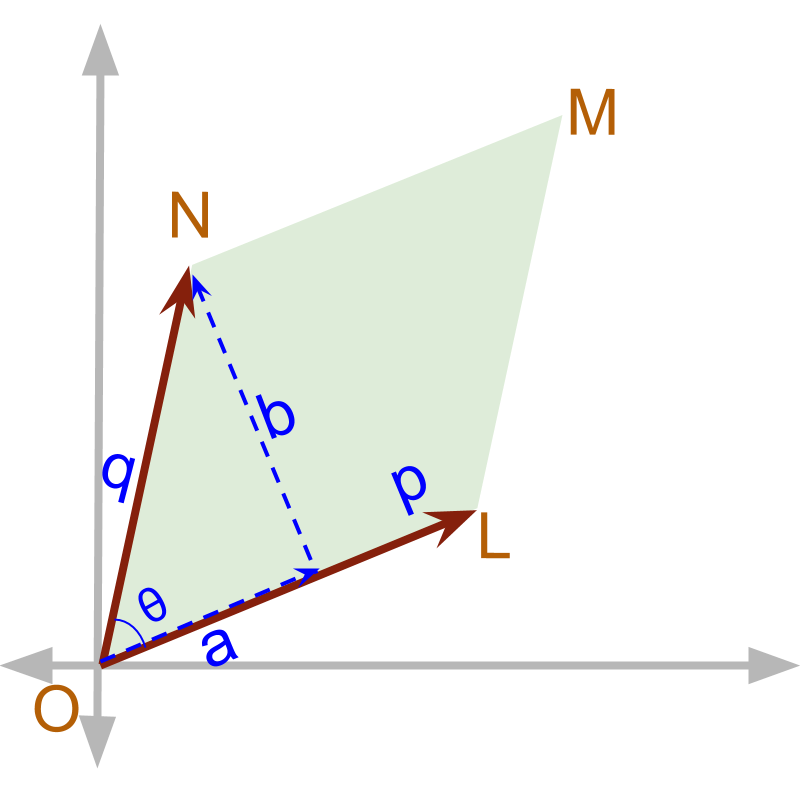maths > vector-algebra

Cross Product : Area of Parallelogram

what you'll learn...

Overview

Vector Cross Product : Area of Parallelogram Form

»  component in perpendicular is calculated by the angle→  $\stackrel{\to }{p}×\stackrel{\to }{q}$$\vec{p} \times \vec{q}$ $=|\stackrel{\to }{p}||\stackrel{\to }{q}|\mathrm{sin}\theta \stackrel{^}{n}$$= | \vec{p} | | \vec{q} | \sin \theta \hat{n}$
→  Note that $|\stackrel{\to }{b}|=|\stackrel{\to }{q}|\mathrm{sin}\theta$$| \vec{b} | = | \vec{q} | \sin \theta$
→  $|\stackrel{\to }{p}||\stackrel{\to }{b}|$$| \vec{p} | | \vec{b} |$ is the area of parallelogram with sides $\stackrel{\to }{p}$$\vec{p}$ and $\stackrel{\to }{q}$$\vec{q}$

parallelogramVector cross product is understood as product between components in perpendicular. In the figure, The magnitude of $\stackrel{\to }{p}×\stackrel{\to }{q}$$\vec{p} \times \vec{q}$ is 'the area of the parallelogram OLMN'.

Area of a parallelogram = base $×$$\times$ height
The base is $|p|$$| p |$ and the height is $|b|$$| b |$.

Two vectors $\stackrel{\to }{p}$$\vec{p}$ and $\stackrel{\to }{q}$$\vec{q}$ with magnitudes $2$$2$ and $3$$3$ respectively are at an angle ${30}^{\circ }$${30}^{\circ}$. What is the area of the parallelogram made by $\stackrel{\to }{p}$$\vec{p}$ and $\stackrel{\to }{q}$$\vec{q}$?

$2×3×{\mathrm{sin}30}^{\circ }$$2 \times 3 \times \sin {30}^{\circ}$
$2×3×\frac{1}{2}$$2 \times 3 \times \frac{1}{2}$
$=3$$= 3$
Area of a Parallelogram made by two vectors: Given $\stackrel{\to }{p}$$\vec{p}$ and $\stackrel{\to }{q}$$\vec{q}$, the area of the parallelogram made by them is
$=|\stackrel{\to }{p}×\stackrel{\to }{q}|$$= | \vec{p} \times \vec{q} |$Vector cross product can be used to find area of the Parallelogram made by two vectors.